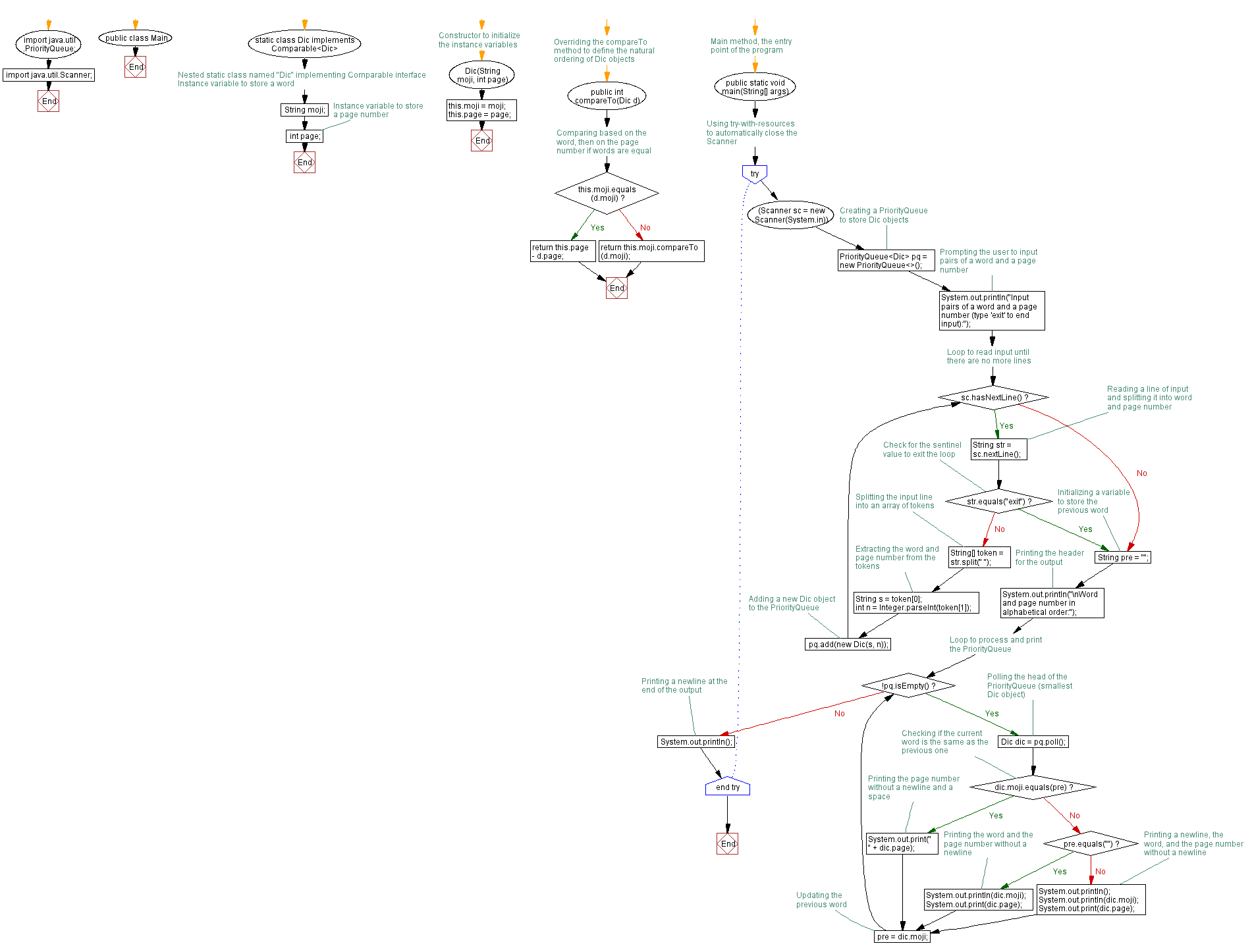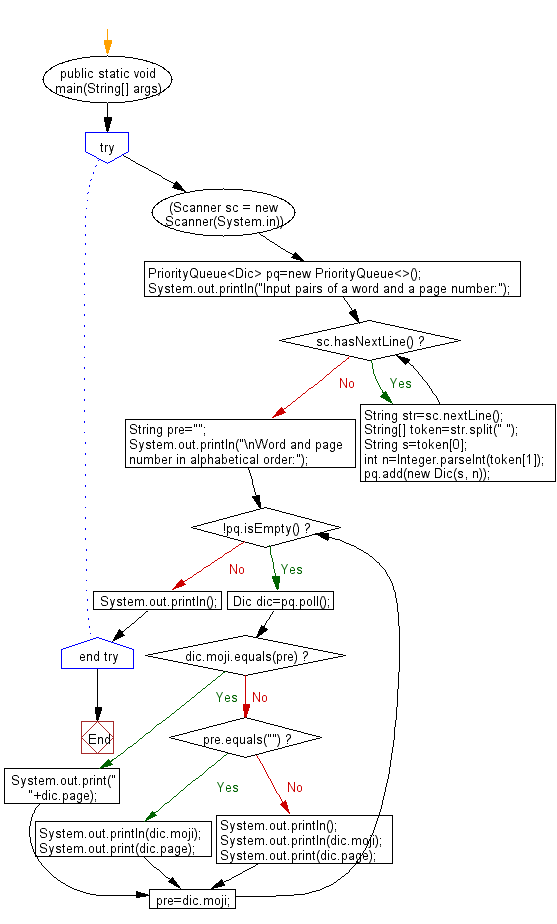# Java Exercises: Reads a list of pairs of a word and a page number, and prints the word and a list of the corresponding page numbers

## Java Basic: Exercise-243 with Solution

Write a Java program which reads a list of pairs of a word and a page number, and prints the word and a list of the corresponding page numbers.
The number of pairs of a word and a page number is less than or equal to 1000. A word never appear in a page more than once. The words should be printed in alphabetical order and the page numbers should be printed in ascending order.

Input:
Input pairs of a word and a page number:
apple 5
banana 6
Word and page number in alphabetical order:
apple
5
banana
6

Sample Solution:

Java Code:

``````import java.util.PriorityQueue;
import java.util.Scanner;

public class Main {

static class Dic implements Comparable<Dic>{
String moji;
int page;
Dic(String moji, int page){
this.moji=moji;
this.page=page;
}
public int compareTo(Dic d) {
if(this.moji.equals(d.moji)) {
return this.page-d.page;
}
else {
return this.moji.compareTo(d.moji);
}
}
}

public static void main(String[] args) {
try(Scanner sc = new Scanner(System.in)){
PriorityQueue<Dic> pq=new PriorityQueue<>();
System.out.println("Input pairs of a word and a page number:");
while(sc.hasNextLine()) {
String str=sc.nextLine();
String[] token=str.split(" ");
String s=token;
int n=Integer.parseInt(token);
}
String pre="";
System.out.println("\nWord and page number in alphabetical order:");
while(!pq.isEmpty()) {
Dic dic=pq.poll();
if(dic.moji.equals(pre)) {
System.out.print(" "+dic.page);
}
else if(pre.equals("")) {
System.out.println(dic.moji);
System.out.print(dic.page);
}
else {
System.out.println();
System.out.println(dic.moji);
System.out.print(dic.page);
}
pre=dic.moji;
}
System.out.println();
}
}
}
```
```

Sample Output:

```Input pairs of a word and a page number:
apple 5
banana 6

Word and page number in alphabetical order:
apple
5
banana
6
```

Flowchart:Flowchart:Java Code Editor: